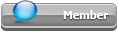## Featured Articles

Check out the latest featured articles.## File Library

Check out the latest downloads available in the File Library.0# Parallel Pump Diaphraghm Pumps With Common Suction Header Design

No replies to this topic
Share this topic:
|

### #1Wise318

Wise318

Brand New Member

•• Members
•• 2 posts

Posted 03 June 2018 - 09:09 AM

Hi,

I would like to get suggestion for calculation of acceleration head in NPSH calculation .

This is positive displacement pump, single acting plunger pump.

with a common header pipe configuration with various pipe dia fron tank to pump

1. Tank source is 3" pipe to common header est 49 m
2. Common header upsized to 4" pipe to pump est 41 m
3. Take off line to each of the pump is 2" pipe est 2 m
4. Pump is 2 "

Scenario 1

How the acceleration losses is calculated ?

Tank Source: 2

Pump running: 12, 11 and 10

Acceleration head, Ha, formula used:

Ha = LVNC/gk, where

L=actual suction pipe length (metres)
V=mean flow velocity in suction line (m/s)
N=pump speed (rpm) stroke per min
C=pump constant factor. I used C=0.4 simplex
g=9.81 m/s2
k=liquid compressibility factor (a constant) 1.4

Total length= 93 m

NPHSHa = 9 m

Q1. Would the acceleration losses be calculated in this summary:

Taking good design design the average velocity is 0.5 m/s which is reasonable low.

L=93m
Vave= 0.5 m/s
N=100
C= 0.4

G.k =9.81 x 1.4 =13.7

LVNC = 1860
Ha =1860/13.7= 135 m

Using this fornula,shows losses greater than the length when the average velocity is considered slow 0.5 m/s.

Deriving this formula
In order to meet the min NPSH, the Ha would need to be < 8 m
( max allowable keeping 1 m npsh margin)

In term of velocity, v

V =(Ha . Gk)/ LNC = 8 x 13.7 / 93 x 100 x 0.4 = 0.029 m/s

The system as followed

https://www.dropbox....23t04/92 Met...

Question 2. May i know if there is any other method to calculate the acceleration head of the system having the system with upsized common header .

Q3 can we considered that the acceleration head for each of the pump only be calculated in the last pipe section from common header to the take off point since the header would be cosidered as main reservoir.

### Similar Topics